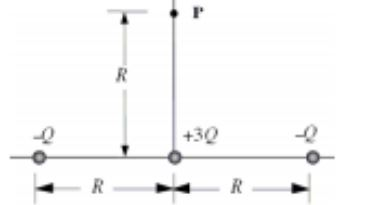# Problem: Three point charges -Q, -Q, and +3Q are arranged along a line as shown in the sketch.Find an expression for the electric potential at the point P in terms of k,R, and Q.

###### FREE Expert Solution

In this problem, we're required to get electric potential.

The electric potential at any point is expressed as:

$\overline{){\mathbit{V}}{\mathbf{=}}\frac{\mathbf{k}\mathbf{Q}}{\mathbf{r}}}$

The total potential is, thus:

83% (171 ratings)###### Problem Details

Three point charges -Q, -Q, and +3Q are arranged along a line as shown in the sketch.

Find an expression for the electric potential at the point P in terms of k,R, and Q.